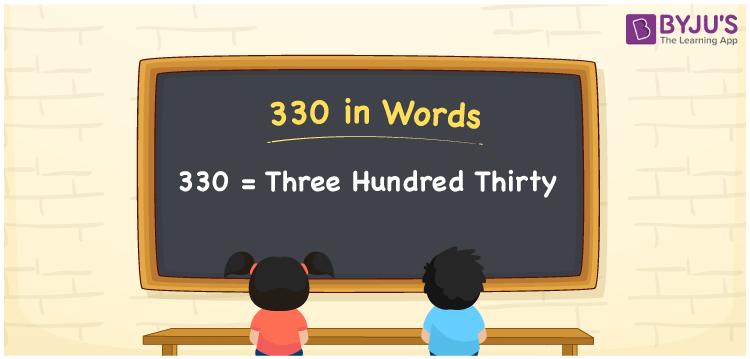# 330 in Words

330 in words is written as “Three Hundred Thirty”. If you give 330 rupees to your friend, then you can say “I have given Rupees Three Hundred Thirty to my friend”. In Mathematics, 330 is a natural number or an integer that is used to represent a value or a quantity. Learn to write the number 330 in words using the place value method and more about Numbers In Words at BYJU’S.

 330 in Words Three Hundred Thirty Three Hundred Thirty in Numbers 330

## 330 in English Words

330 in Words using English alphabets is expressed as given below:## How to Write 330 in Words?

330 in words is written based on the position of each digit in the given number. Since 330 is a three-digit number, thus the digits can be represented as:

 Hundreds Tens Ones 3 3 0

From the above table, we can see,

• 3 is at Hundreds
• 3 is at Tens
• 0 is at Ones

We need to read the number from right to left, in the table. Hence, it is spelt as Three Hundred Thirty.

### Expanded Form of 330

We can write the expanded form of 330 as:

3 at Hundreds + 3 at Tens + 0 at Ones

= 3 × 100 + 3 × 10 + 0 × 1

= 300 + 30 + 0

= 330

= Three Hundred Thirty

330 is a whole number that is succeeded by 329 and preceded by 331. Find more about the number 330 below:

• 330 in Words – Three Hundred Thirty
• Is 330 an odd number? – No
• Is 330 an even number? – Yes
• Is 330 a perfect square number? – No
• Is 330 a perfect cube number? – No
• Is 330 a prime number? – No
• Is 330 a composite number? – Yes

## Frequently Asked Questions on 330 in words

Q1

### What is 330 in words?

330 in words is given by Three Hundred Thirty.
Q2

### What is the rule to write 330 in words?

330 in words is written based on the place values of each digit.

3 → Hundreds

3 → Tens

0 → Ones

Thus, the number is read as Three Hundred Thirty.

Q3

### How to spell 330 in English words?

330 in English is spelt as Three Hundred Thirty.